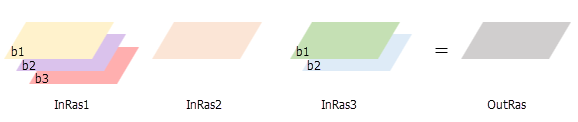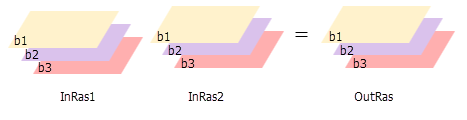# Cell Statistics (Spatial Analyst)

## Summary

Calculates a per-cell statistic from multiple rasters.

The available statistics are Majority, Maximum, Mean, Median, Minimum, Minority, Percentile, Range, Standard deviation, Sum, and Variety.

## Usage

• The order of the input rasters is irrelevant for this tool.

• For the Maximum, Minimum, Mean, Median, Majority, Minority, Percentile, and Sum statistic types, if a single raster is used as the input, the output cell values will be the same as the input cell values. For Range and Standard deviation, the output cell values will all be 0. For Variety, it will be 1.

• If the Process as multiband parameter is unchecked (process_as_multiband is set to SINGLE_BAND in Python), each band from a multiband raster input will be processed separately as a single band raster, and the output will be a single band raster.• If the Process as multiband parameter is checked (process_as_multiband is set to MULTI_BAND in Python), each multiband raster input will be processed as a multiband raster, and the output will be a multiband raster. The output raster will also be multiband if the inputs are a combination of a multiband raster and constants. The number of bands in each multiband input must be the same.

The tool will perform the operation on each band from one input using the corresponding band from the other input. If one of the inputs is a multiband raster and the other input is a constant, the tool will perform the operation using the constant value for each band in the multiband input.• In ArcPy, if all inputs are multidimensional rasters with the same number of variables, the tool will perform the operation for all slices with the same dimension value, and the output will be a multidimensional raster. The variables in the inputs must have the same dimensions or common dimensions.

If all of the inputs are multidimensional rasters with one variable but different names, set the matchMultidimensionalVariable geoprocessing environment to False to perform the operation.

In ArcPy, if one or more of the inputs is a multidimensional raster and the other input is a constant, the tool will perform the operation for all slices for all variables using the constant value, and the output will be a multidimensional raster.

• See Analysis environments and Spatial Analyst for additional details on the geoprocessing environments that apply to this tool.

## Parameters

 Label Explanation Data Type Input rasters or constant values A list of input rasters for which a statistic will be calculated for each cell in the Analysis window.A number can be used as an input; however, the cell size and extent must first be set in the environment.If the Process as multiband parameter is checked, all multiband inputs must have an equal number of bands. Raster Layer; Constant Overlay statistic(Optional) Specifies the statistic type to be calculated.The default statistic type is Mean.Mean—The mean (average) of the inputs will be calculated. This is the default.Majority—The majority (value that occurs most often) of the inputs will be determined.Maximum—The maximum (largest value) of the inputs will be determined.Median—The median of the inputs will be calculated.Minimum—The minimum (smallest value) of the inputs will be determined.Minority—The minority (value that occurs least often) of the inputs will be determined.Percentile—The percentile of the inputs will be calculated. The 90th percentile is calculated by default. You can specify other values (from 0 to 100) using the Percentile value parameter.Range—The range (difference between largest and smallest value) of the inputs will be calculated.Standard deviation—The standard deviation of the inputs will be calculated.Sum—The sum (total of all values) of the inputs will be calculated.Variety—The variety (number of unique values) of the inputs will be calculated. String Ignore NoData in calculations(Optional) Specifies whether NoData values will be ignored by the statistic calculation.Checked—At the processing cell location, if any of the input rasters has NoData, that NoData value will be ignored. The statistics will be computed by only considering the cells with valid data. This is the default.Unchecked—If the processing cell location for any of the input rasters is NoData, the output for that cell will be NoData. Boolean Process as multiband(Optional) Specifies how the input multiband raster bands will be processed. Unchecked—Each band from a multiband raster input will be processed separately as a single band raster. This is the default.Checked—Each multiband raster input will be processed as a multiband raster. The operation will be performed for each band from one input using the corresponding band number from the other inputs. Boolean Percentile value(Optional) The percentile to calculate. The default is 90, indicating the 90th percentile.The values can range from 0 to 100. The 0th percentile is essentially equivalent to the minimum statistic, and the 100th percentile is equivalent to maximum. A value of 50 will produce essentially the same result as the median statistic.This option is only available if the Overlay Statistic parameter is set to Percentile. Double Percentile interpolation type(Optional) Specifies the method of interpolation to be used when the specified percentile value lies between two input cell values.Auto-detect—If the input rasters are of integer pixel type, the Nearest method is used. If the input rasters are of floating point pixel type, the Linear method is used. This is the default.Nearest—The nearest available value to the desired percentile is used. In this case, the output pixel type is the same as that of the input rasters.Linear—The weighted average of the two surrounding values from the desired percentile is used. In this case, the output pixel type is floating point. String

### Return Value

 Label Explanation Data Type Output raster The output raster.For each cell, the value is determined by applying the specified statistic type to the input rasters at that location. Raster

`CellStatistics(in_rasters_or_constants, {statistics_type}, {ignore_nodata}, {process_as_multiband}, {percentile_value}, {percentile_interpolation_type})`
 Name Explanation Data Type in_rasters_or_constants[in_raster_or_constant,...] A list of input rasters for which a statistic will be calculated for each cell in the Analysis window.A number can be used as an input; however, the cell size and extent must first be set in the environment.If the processing_as_multiband parameter is set to MULTI_BAND, all multiband inputs should have an equal number of bands. Raster Layer; Constant statistics_type(Optional) Specifies the statistic type to be calculated.MEAN—The mean (average) of the inputs will be calculated. This is the default.MAJORITY—The majority (value that occurs most often) of the inputs will be determined.MAXIMUM—The maximum (largest value) of the inputs will be determined.MEDIAN—The median of the inputs will be calculated.MINIMUM—The minimum (smallest value) of the inputs will be determined.MINORITY—The minority (value that occurs least often) of the inputs will be determined.PERCENTILE—The percentile of the inputs will be calculated. The 90th percentile is calculated by default. You can specify other values (from 0 to 100) using the percentile_value parameter.RANGE—The range (difference between largest and smallest value) of the inputs will be calculated.STD—The standard deviation of the inputs will be calculated.SUM—The sum (total of all values) of the inputs will be calculated.VARIETY—The variety (number of unique values) of the inputs will be calculated.The default statistic type is MEAN. String ignore_nodata(Optional) Specifies whether NoData values will be ignored by the statistic calculation.DATA—At the processing cell location, if any of the input rasters has NoData, that NoData value will be ignored. The statistics will be computed by only considering the cells with valid data. This is the default.NODATA—If the processing cell location for any of the input rasters is NoData, the output for that cell will be NoData. Boolean process_as_multiband(Optional) Specifies how the input multiband raster bands will be processed. SINGLE_BAND—Each band from a multiband raster input will be processed separately as a single band raster. This is the default.MULTI_BAND—Each multiband raster input will be processed as a multiband raster. The operation will be performed for each band from one input using the corresponding band number from the other inputs. Boolean percentile_value(Optional) The percentile to calculate. The default is 90, indicating the 90th percentile.The values can range from 0 to 100. The 0th percentile is essentially equivalent to the minimum statistic, and the 100th percentile is equivalent to maximum. A value of 50 will produce essentially the same result as the median statistic.This option is only supported if the statistics_type parameter is set to PERCENTILE. Double percentile_interpolation_type(Optional) Specifies the method of interpolation to be used when the specified percentile value lies between two input cell values.AUTO_DETECT—If the input rasters are of integer pixel type, the NEAREST method is used. If the input rasters are of floating point pixel type, the LINEAR method is used. This is the default.NEAREST—The nearest available value to the desired percentile is used. In this case, the output pixel type is the same as that of the input rasters.LINEAR—The weighted average of the two surrounding values from the desired percentile is used. In this case, the output pixel type is floating point. String

### Return Value

 Name Explanation Data Type out_raster The output raster.For each cell, the value is determined by applying the specified statistic type to the input rasters at that location. Raster

### Code sample

CellStatistics example 1 (Python window)

This example calculates the standard deviation per cell on several input Grid rasters and outputs the result as an IMG raster.

``````import arcpy
from arcpy import env
from arcpy.sa import *
env.workspace = "C:/sapyexamples/data"
outCellStats = CellStatistics(["degs", "negs", "cost"], "STD", "DATA")
outCellStats.save("C:/sapyexamples/output/outcellstats.img")``````
CellStatistics example 2 (stand-alone script)

This example calculates the range per cell per band on several input multiband rasters and creates a multiband output raster.

``````# Name: CellStatistics_Ex_standalone.py
# Description: Calculates a per-cell statistic from multiple multiband rasters
#               and process as multiband.
# Requirements: Spatial Analyst Extension

# Import system modules
import arcpy
from arcpy.sa import *

# Check out the ArcGIS Spatial Analyst extension license
arcpy.CheckOutExtension("Spatial")

# Set the analysis environments
arcpy.env.workspace = "C:/sapyexamples/data"

# Set the local variables
inRaster01 = "degs_MB"
inRaster02 = "negs_MB"
inRaster03 = "cost_MB"

# Execute CellStatistics
outCellStatistics = CellStatistics([inRaster01, inRaster02, inRaster03], "RANGE", "NODATA", "MULTI_BAND")

# Save the output
outCellStatistics.save("C:/sapyexamples/output/cellstats_MB.tif")``````

## Licensing information

• Basic: Requires Spatial Analyst or Image Analyst
• Standard: Requires Spatial Analyst or Image Analyst
• Advanced: Requires Spatial Analyst or Image Analyst